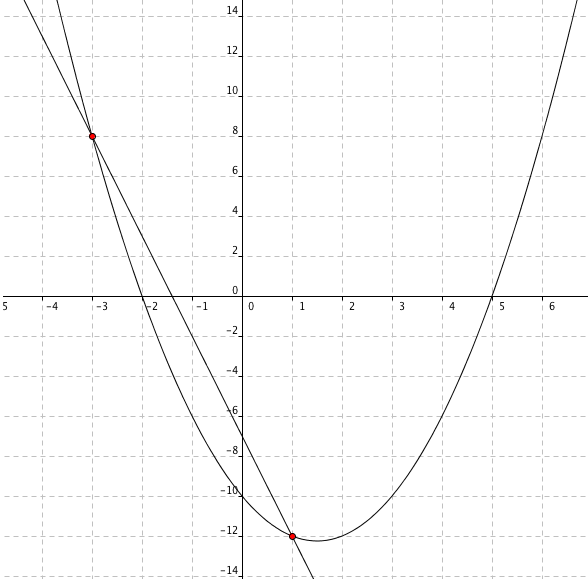### Home > CAAC > Chapter 12 > Lesson 12.1.3 > Problem12-30

12-30.

Graph the two functions below and find all points where they intersect. List all points in the form (x, y). Homework Help ✎

f(x) = x2 − 3x − 10
g(x) = −5x − 7What are the two intersecting points?